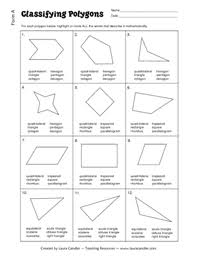Mathematic

# Analysis on Classifying PolygonsGeneral objective of this article is to Analysis on Classifying Polygons. Here explain Classifying Polygons in Geometry term. A polygon can be defined as a closed planar figure that is the union of a finite number of line segments. In this definition, you consider closed as an undefined term. The term polygon is derived from a Greek word meaning “many‐angled.” Polygons first fit into two general categories: convex and not convex.